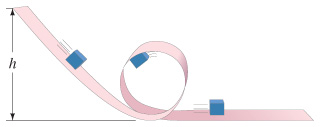# Problem: The small mass m sliding without friction along the looped track shown in the figure is to remain on the track at all times, even at the very top of the loop of radius r.If the actual release height is 4 h, calculate the normal force exerted by the track at the bottom of the loop.If the actual release height is 4 h, calculate the normal force exerted by the track at the top of the loop.If the actual release height is 6 h, calculate the normal force exerted by the track after the block exits the loop onto the flat section.Determine the minimum release height h.

###### FREE Expert Solution
97% (230 ratings)
###### Problem Details

The small mass m sliding without friction along the looped track shown in the figureis to remain on the track at all times, even at the very top of the loop of radius r.

If the actual release height is 4 h, calculate the normal force exerted by the track at the bottom of the loop.

If the actual release height is 4 h, calculate the normal force exerted by the track at the top of the loop.

If the actual release height is 6 h, calculate the normal force exerted by the track after the block exits the loop onto the flat section.

Determine the minimum release height h.

Frequently Asked Questions

What scientific concept do you need to know in order to solve this problem?

Our tutors have indicated that to solve this problem you will need to apply the Motion Along Curved Paths concept. You can view video lessons to learn Motion Along Curved Paths. Or if you need more Motion Along Curved Paths practice, you can also practice Motion Along Curved Paths practice problems.